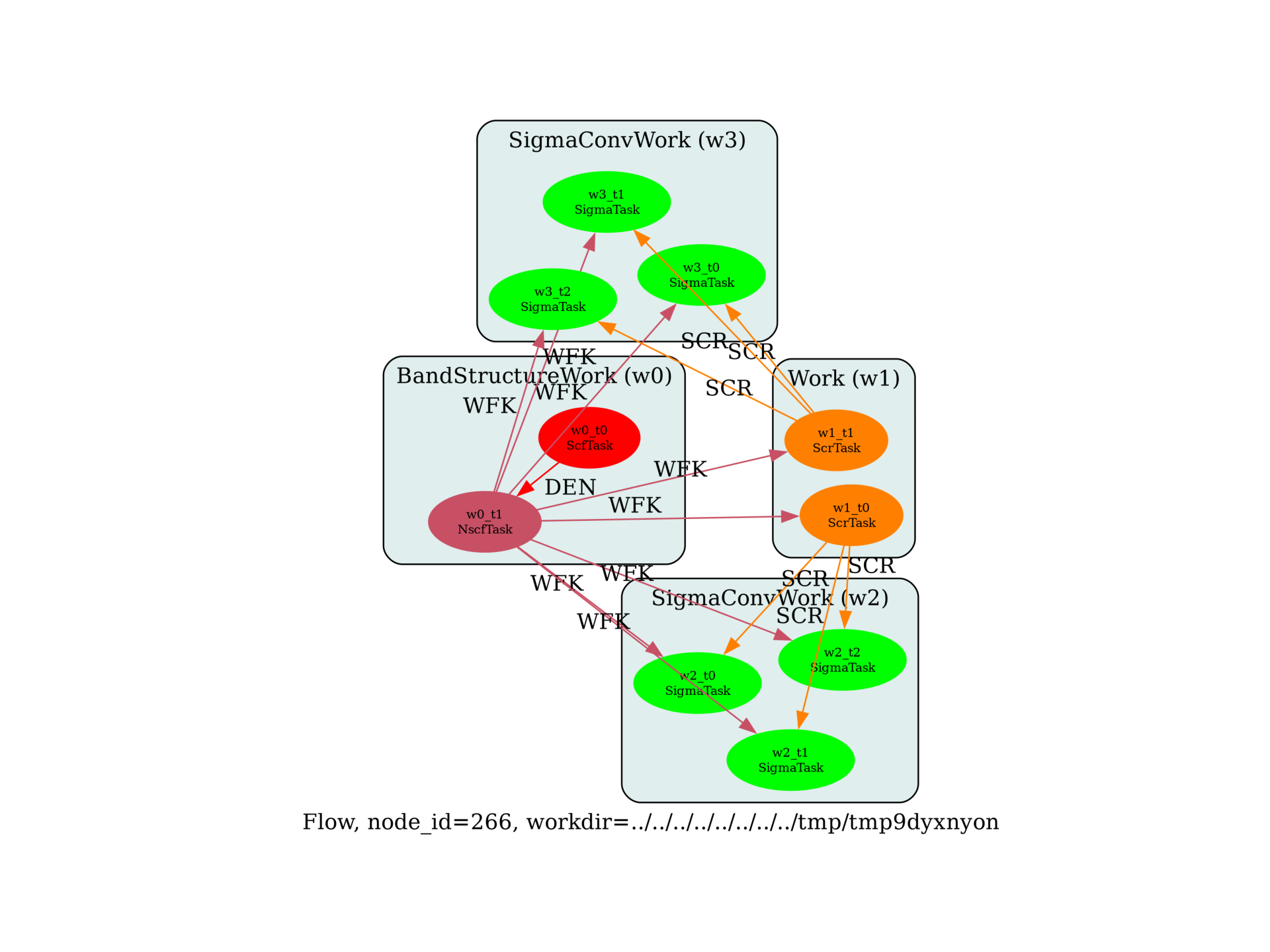# G0W0 convergence study¶

G0W0 convergence study wrt ecuteps and the number of bands in W.Out:

/Users/gmatteo/git_repos/pymatgen/pymatgen/util/plotting.py:550: UserWarning: Matplotlib is currently using agg, which is a non-GUI backend, so cannot show the figure.
plt.show()


import sys
import os
import numpy as np

import abipy.abilab as abilab
import abipy.data as abidata
import abipy.flowtk as flowtk

def make_inputs(paral_kgb=1):
"""
Returns a tuple of 4 input files for SCF, NSCF, SCR, SIGMA calculations.
These files are then used as templates for the convergence study
wrt ecuteps and the number of bands in W.
"""
multi = abilab.MultiDataset(abidata.structure_from_ucell("SiC"),
pseudos=abidata.pseudos("14si.pspnc", "6c.pspnc"), ndtset=4)

ecut = 12
global_vars = dict(
ecut=ecut,
istwfk="*1",
paral_kgb=paral_kgb,
gwpara=2,
iomode=1,
)

ecuteps = 4
ngkpt = [4, 4, 4]
shiftk = [0, 0, 0]

multi.set_vars(global_vars)
multi.set_kmesh(ngkpt=ngkpt, shiftk=shiftk)

# SCF
multi.set_vars(
nband=10,
tolvrs=1.e-8,
)

# NSCF
multi.set_vars(
nband=25,
tolwfr=1.e-8,
iscf=-2
)

# SCR
multi.set_vars(
optdriver=3,
ecutwfn=ecut,
nband=20,
symchi=1,
inclvkb=0,
ecuteps=ecuteps,
)

# SIGMA
multi.set_vars(
optdriver=4,
nband=20,
ecutwfn=ecut,
ecutsigx=ecut,
#ecutsigx=(4*ecut), ! This is problematic
symsigma=1,
ecuteps=ecuteps,
)

multi.set_kptgw(kptgw=[[0,0,0], [0.5, 0, 0]], bdgw=[1, 8])

return multi.split_datasets()

def build_flow(options):
# Working directory (default is the name of the script with '.py' removed and "run_" replaced by "flow_")
if not options.workdir:
options.workdir = os.path.basename(sys.argv).replace(".py", "").replace("run_", "flow_")

# Get our templates
scf_inp, nscf_inp, scr_inp, sig_inp = make_inputs()

ecuteps_list = np.arange(2, 8, 2)
max_ecuteps = max(ecuteps_list)

flow = flowtk.Flow(workdir=options.workdir, manager=options.manager)

# Band structure work to produce the WFK file
bands = flowtk.BandStructureWork(scf_inp, nscf_inp)
flow.register_work(bands)

# Build a work made of two SCR runs with different value of nband
# Use max_ecuteps for the dielectric matrix (sigma tasks will
# read a submatrix when we test the convergence wrt to ecuteps.
scr_work = flowtk.Work()

for inp in scr_inp.generate(nband=[10, 15]):
inp.set_vars(ecuteps=max_ecuteps)

flow.register_work(scr_work)

# Do a convergence study wrt ecuteps, each work is connected to a
# different SCR file computed with a different value of nband.

# Build a list of sigma inputs with different ecuteps
sigma_inputs = list(sig_inp.generate(ecuteps=ecuteps_list))

flow.register_work(sigma_conv)

return flow

# This block generates the thumbnails in the AbiPy gallery.
# You can safely REMOVE this part if you are using this script for production runs.
__name__ = None
import tempfile
options = flowtk.build_flow_main_parser().parse_args(["-w", tempfile.mkdtemp()])
build_flow(options).graphviz_imshow()

@flowtk.flow_main
def main(options):
"""
This is our main function that will be invoked by the script.
flow_main is a decorator implementing the command line interface.
Command line args are stored in options.
"""
return build_flow(options)

if __name__ == "__main__":
sys.exit(main())


Total running time of the script: ( 0 minutes 1.427 seconds)

Gallery generated by Sphinx-Gallery